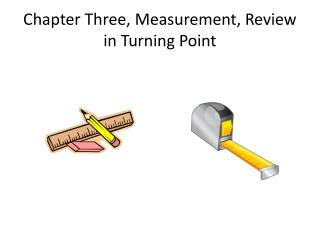Download PresentationChapter Three, Measurement, Review in Turning Point

# Chapter Three, Measurement, Review in Turning Point

Download Presentation## Chapter Three, Measurement, Review in Turning Point

- - - - - - - - - - - - - - - - - - - - - - - - - - - E N D - - - - - - - - - - - - - - - - - - - - - - - - - - -
##### Presentation Transcript

1. Chapter Three, Measurement, Review in Turning Point

2. 4. It takes 38 stickers to fill a page. Charlene has 14 stickers.Which number sentence shows how many more stickers she needs to fill the page? A. ?+8= 14 B. 14+? =38 C. 14+38=? index

3. 17. The rectangular arrays show a number fact. Which number fact do they show? • 4 × 6 = 6 × 4 • 4 + 6 = 6 + 4 • 4 ÷ 6 = 6 ÷ 4 index

4. 27. Which object is about 10 feet high? • A desk • A classroom wall • A mountain index

5. 38. Which number makes this number sentence true? ? + 3 = 15 A. 5 B. 12 C. 18 index

6. 44. When would you use liters? • to find the weight of a book • to find the length of a pencil • to find the volume of a bottle Index

7. 46. Use your inch ruler to measure each side of this rectangle.Write the length of each side of the rectangle on the blank lines. Write the answer on separate paper. Scoring Guidelines index

8. 1. Which unit is best used for measuring the amount of water in a swimming pool? • Ounce • Foot • Gallon Index

9. 3. About how long is a pencil? • 6 inches • 6 feet • 6 yards Index

10. 8. Rachel lines up beans on two sides of a rectangle. • 7 • 13 • 20 How many beans will Rachel need to go all the way around the rectangle? Index

11. 18. A tomato plant is 7 inches tall. It grows 2 inches each week. How tall will the tomato plant be at the end of 3 weeks? • 9 inches • 10 inches • 13 inches Index

12. 40. Which number makes this statement true? • 5 • 21 • 84 _?_ - 8 = 13 Index

13. 45. Carole has 7 marbles. Ezra gives her 3 marbles. Tonya gave Carole 2 more marbles. The total number of marbles that Carole has can be shown by (7 + 3) + 2. This is the same as: • 7 + (3 + 2) • (7 + 3) + (7 + 2) • (7 + 3) + (3 + 2) Index

14. 46. Monica had 18 books. She shared some books with a friend and had 12 books left.Which number sentence could be used to find the number of books Monica shared? A. 18 – n = 12 B. 12 – 18 = n C. 18 + 12 = n Index

15. 3. Which number makes this number sentence true? • 7 • 14 • 35 Index

16. 12. Laura baked 36 cookies. Her brother Sam ate n cookies. Which expression represents the number of cookies Laura has left? • n – 36 • 36 – n • 36 ÷ n Index

17. 27. About how many paper clips long is this line? • 2 • 4 • 6 Index

18. 30. About how much does a spoon weigh? • 50 meters • 50 grams • 50 kilograms Index

19. 44. Which object would hold about one gallon of water? • A • B • C A B C Index

20. 6. About how many quarts of liquid are in this pitcher? • 1 ½ quarts • 2 quarts • 2 ½ quarts index

21. 8. Which number makes this statement true? 18 + ? < 22 • 3 • 8 • 14 index

22. 33. Pam is cutting squares of colored paper to glue together. She wants the squares to be the same size. She made a mistake and cut the square shown. 3 cm She wanted each side to be 3 inches long. Draw the square that Pam wanted to cut. Write the answer on separate paper. Scoring Guidelines index

23. 19. Which unit is best for measuring the length of your classroom? • inches • feet • miles index

24. 3. Which number makes this number sentence true?7 + ? = 32 A. 15 B. 25 C. 39 Index

25. 7. Which thermometer shows 77ºF? A. B. C. Index

26. 12. Which table has three pairs of values that make this number sentence true?? + 5 = ? A. B. C. Index

27. 25. Use your ruler to draw a rectangle with sides 4 cm and 7 cm.Label the length of each side. Write the answer on separate paper. Index Scoring Guidelines

28. 31. Which is best measured in ounces? A. length of a pencil B. weight of a book C. time on the clock Index